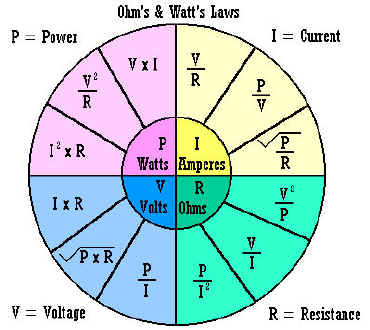## Ohm’s Law 101

Through experiments circa 1828, George Simon Ohm determined that the amount of current, symbol is I, in a circuit depends on its resistance, symbol is R , and the line voltage V.

Hence Ohm’s Law states, I = V/R

If any two of the variables I, V, R are known, the third can be calculated.

Note that a low voltage value with a low resistance level will produce a large current flow. And with a high voltage value, the current flow can be low if there is a large level of resistance in the circuit.

The three forms of Ohm’s law are I = V/R; V = I x R; R = V/I

Additionally, according to Ohm’s Law, the amount of power consumed in a circuit is the product of current and voltage.

P = V x I

Similarly, if any two of the variables P, I, V are known, the third can be calculated.

These relationships apply to both alternating and direct current:One ampere is the current flow produced by one volt of potential difference across one ohm of resistance level.

 Practical Units of Electricity Coulomb Ampere Volt Watt Ohm Siemens 6.25 x 1018 electrons Coulomb / second Joule / coulomb Joule / second Volt / Amepere Ampere / volt

Units of measurement:    I - amperes (amps),   V - volts,   R - ohms,   P - watts

 Basic Unit Prefixes Prefix Symbol Power of micro µ 0.000,001 = 1 x 10-6 milli m 1,000 = 1 x 10-3 kilo k 1,000 = 1 x 103 mega M 1,000,000 = 1 x 106

https://analyzedetectnetwork.com